# NCERT Solutions, Matrices, Part - 2, Class 12, Maths Notes - Class 12

## Class 12: NCERT Solutions, Matrices, Part - 2, Class 12, Maths Notes - Class 12

The document NCERT Solutions, Matrices, Part - 2, Class 12, Maths Notes - Class 12 is a part of Class 12 category.
All you need of Class 12 at this link: Class 12

Matrices and Determinants

Exercise 3.2
Question 1:

Let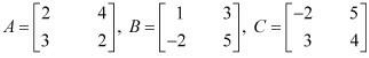Find each of the following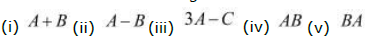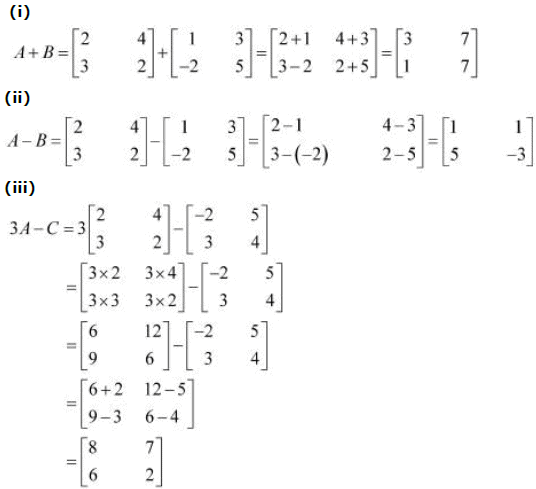(iv) Matrix A has 2 columns. This number is equal to the number of rows in matrix B.
Therefore, AB is defined as: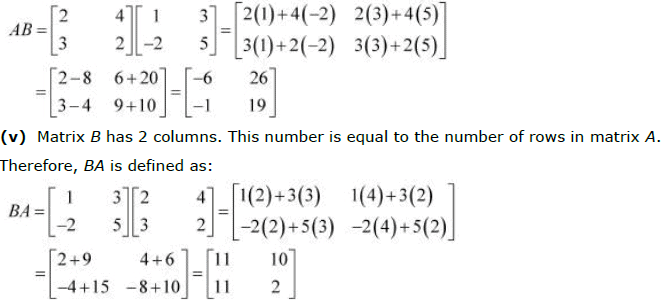Question 2: Compute the following: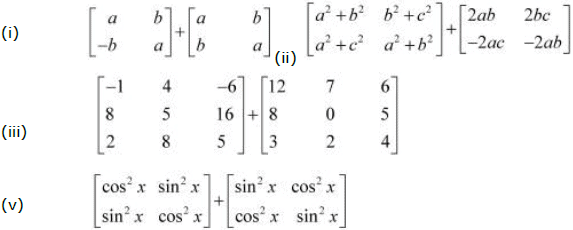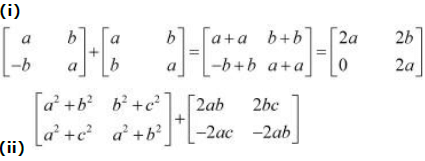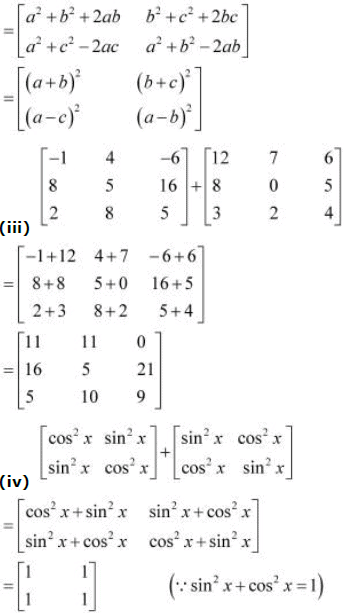Question 3: Compute the indicated products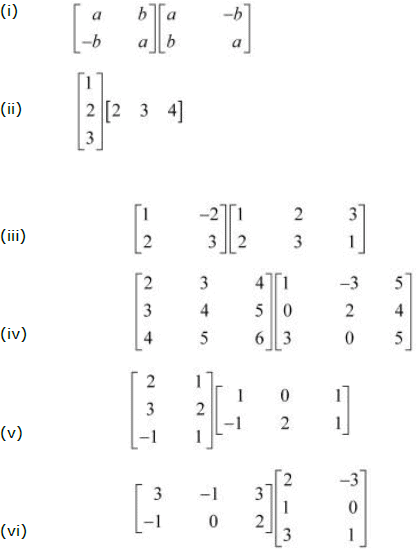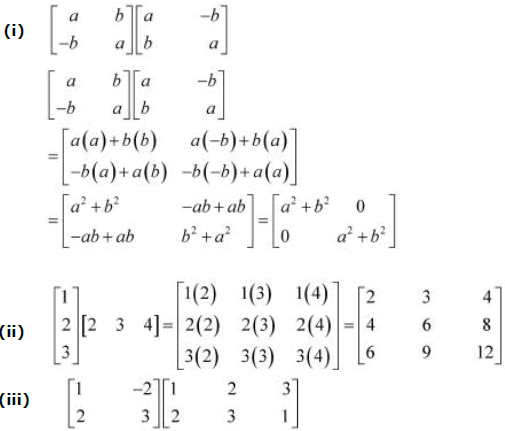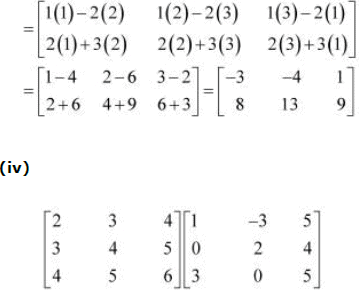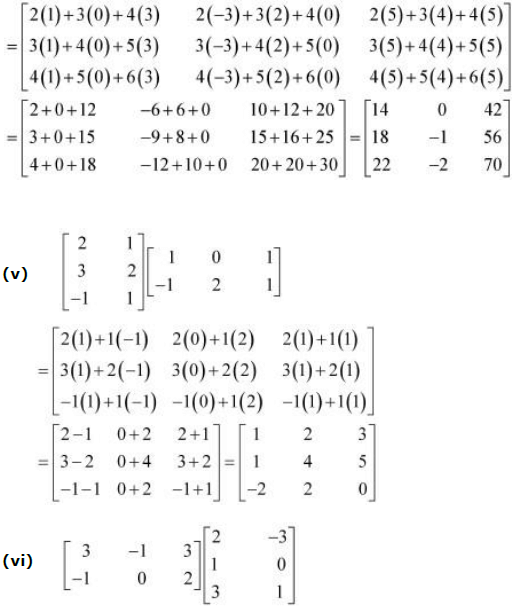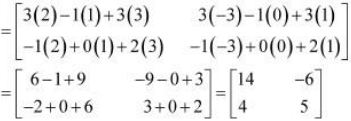Question 4: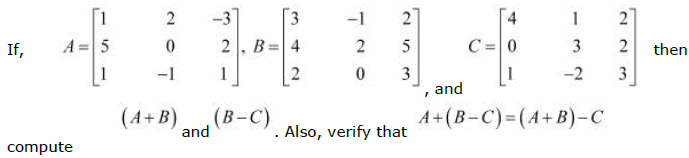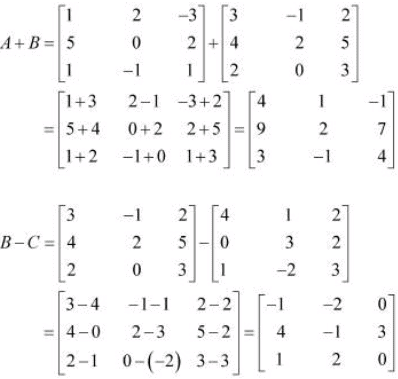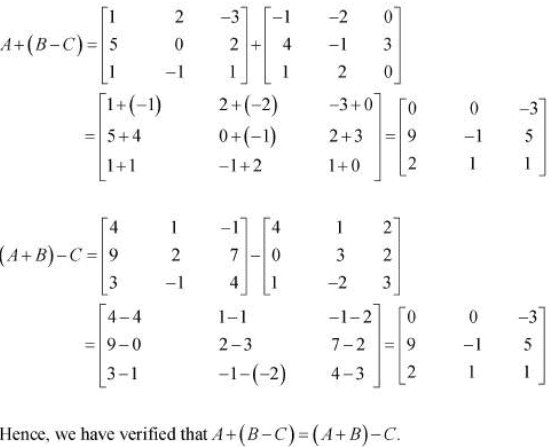Question 5: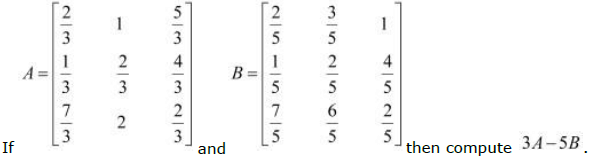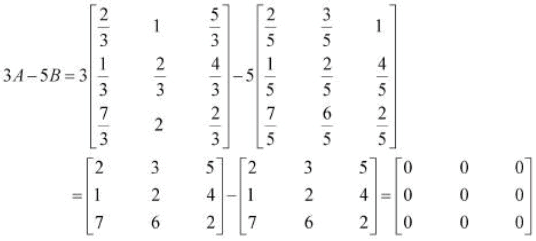Question 6: Simplify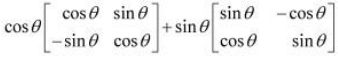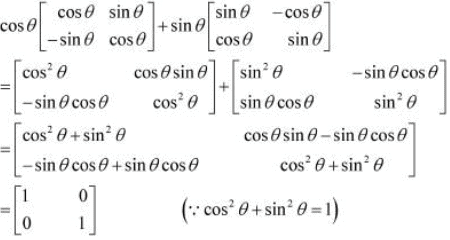Question 7: Find X and Y, if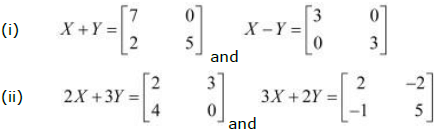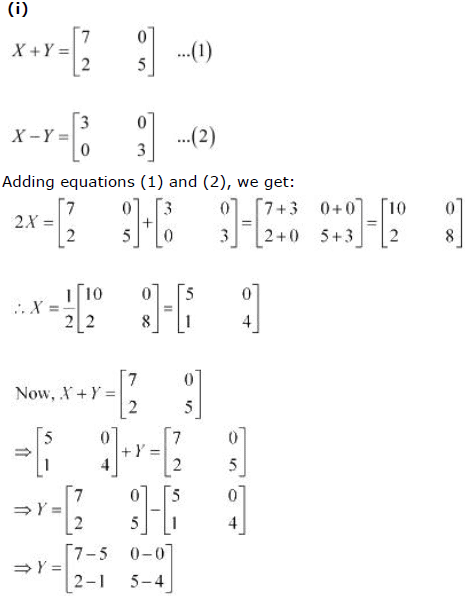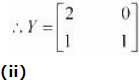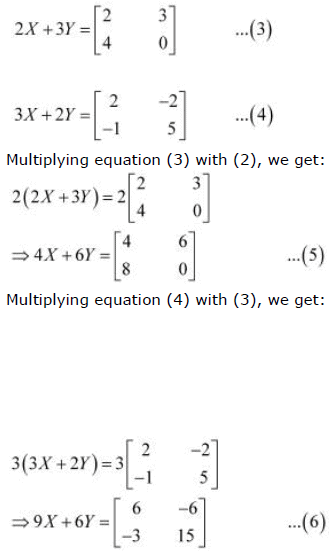From (5) and (6), we have: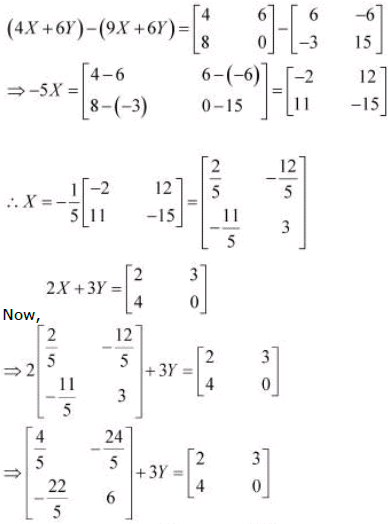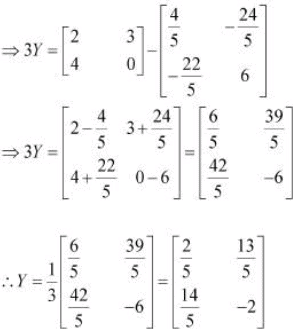Question 8: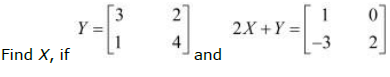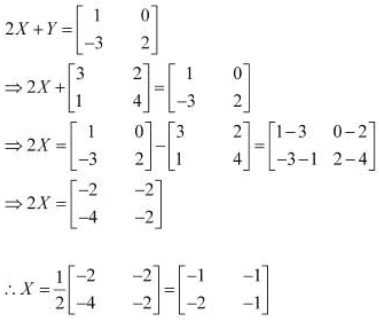Question 9:
Find x and y, if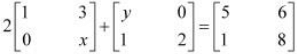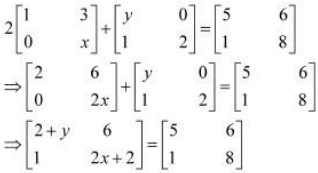Comparing the corresponding elements of these two matrices, we have: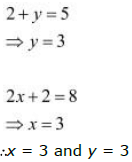Question 10: Solve the equation for x, y, z and t if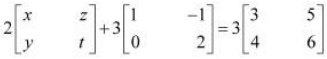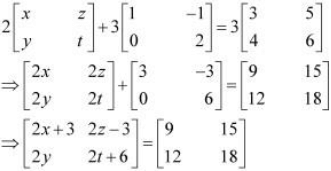Comparing the corresponding elements of these two matrices, we get: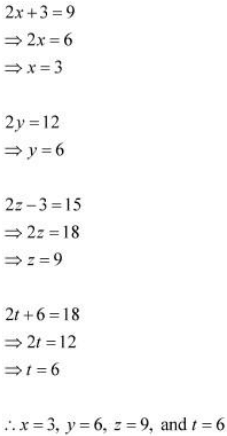Question 11:
If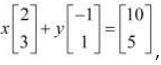, find values of x and y.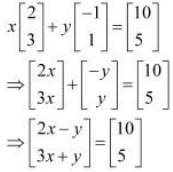Comparing the corresponding elements of these two matrices, we get:
2x − y = 10 and 3x + y = 5
Adding these two equations, we have:
5x = 15
⇒ x = 3
Now, 3x + y = 5
⇒ y = 5 − 3x
⇒ y = 5 − 9 = −4
∴x = 3 and y = −4

Question 12:
Given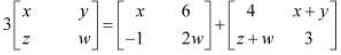, find the values of x, y, z and w.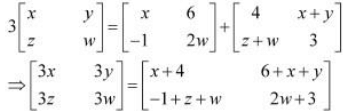Comparing the corresponding elements of these two matrices, we get: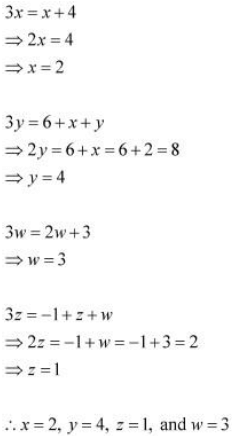Question 13:
If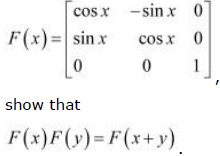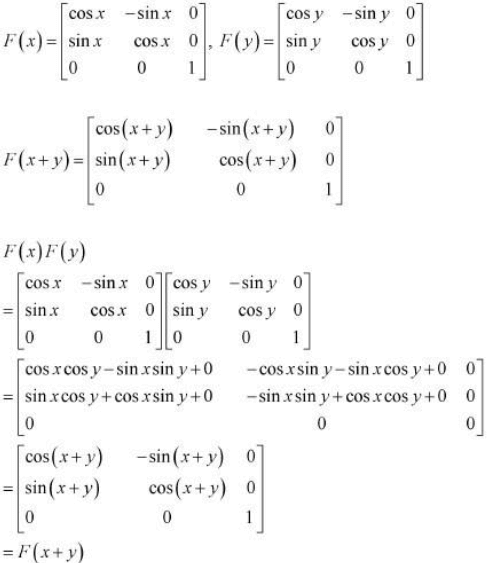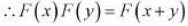Question 14:
Show that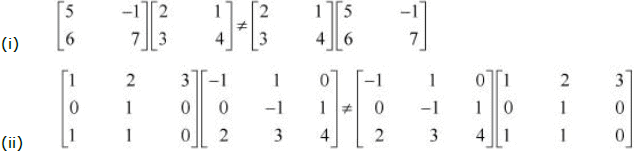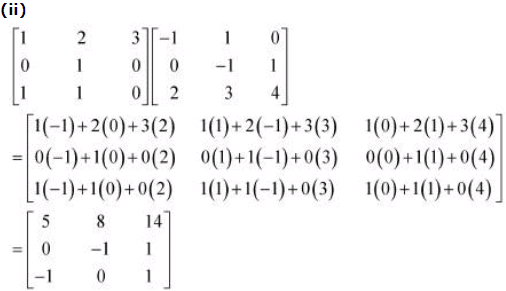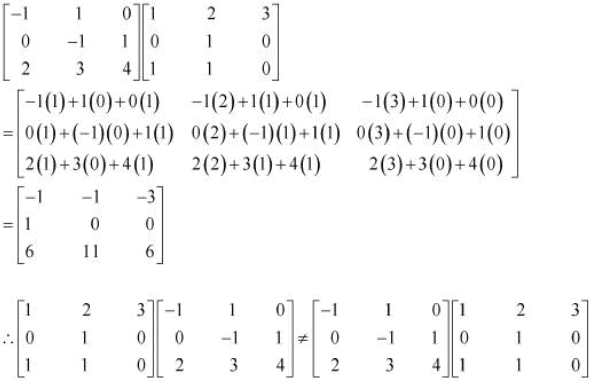Question 15: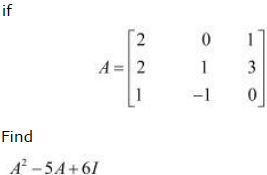We have A2 = A × A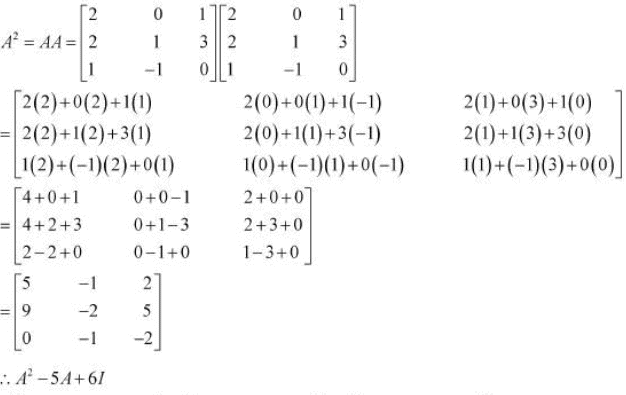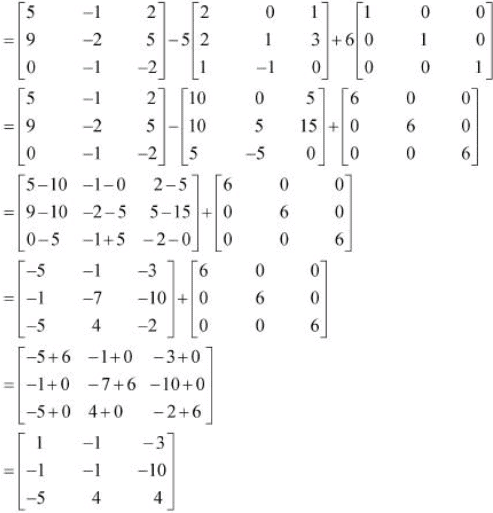Question 16: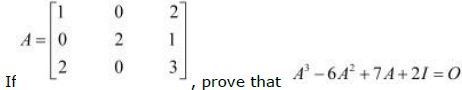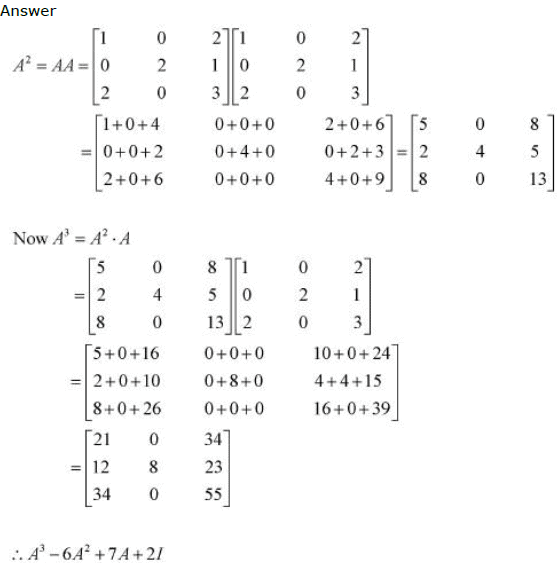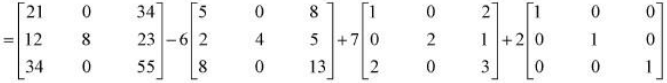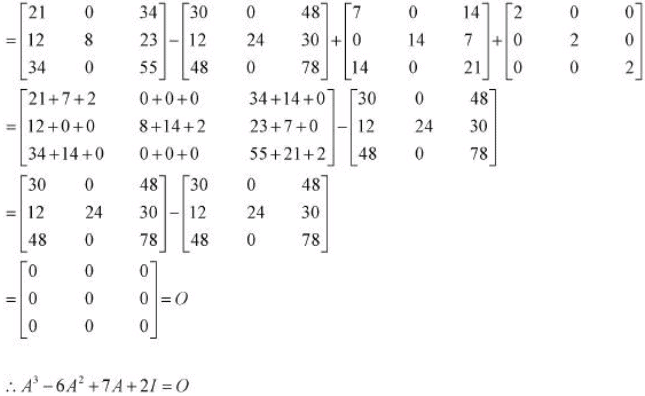Question 17: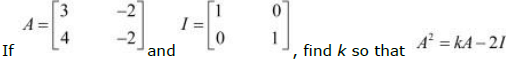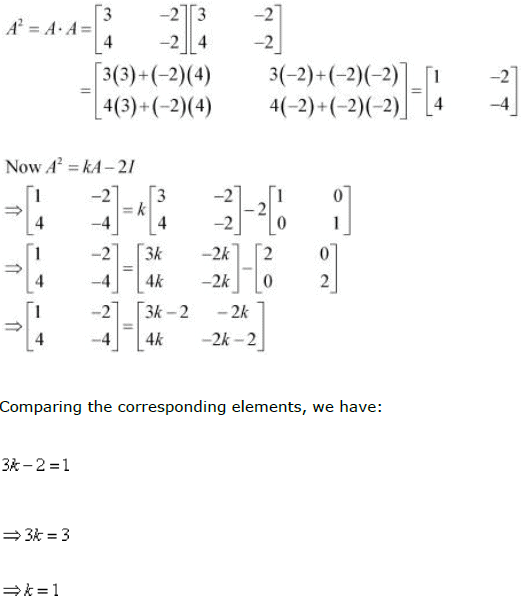Thus, the value of k is 1.

Question 18: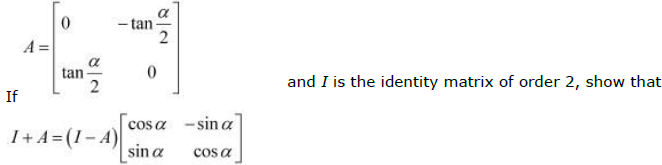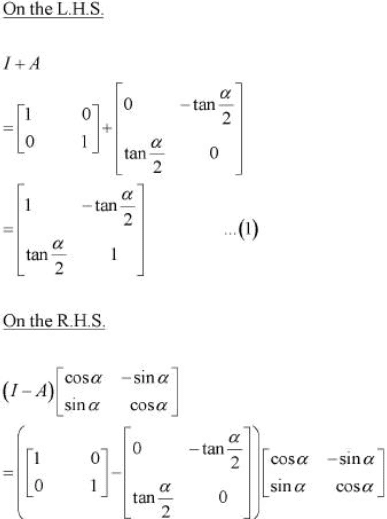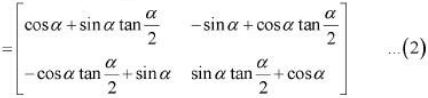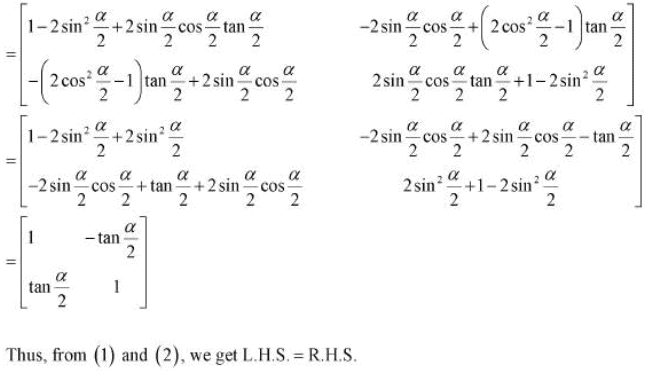Question 19:
A trust fund has Rs 30,000 that must be invested in two different types of bonds. The first
bond pays 5% interest per year, and the second bond pays 7% interest per year. Using
matrix multiplication, determine how to divide Rs 30,000 among the two types of bonds.
If the trust fund must obtain an annual total interest of:
(a) Rs 1,800

(b) Rs 2,000

(a) Let Rs x be invested in the first bond. Then, the sum of money invested in the second
bond will be Rs (30000 − x).
It is given that the first bond pays 5% interest per year and the second bond pays 7%
interest per year.
Therefore, in order to obtain an annual total interest of Rs 1800, we have: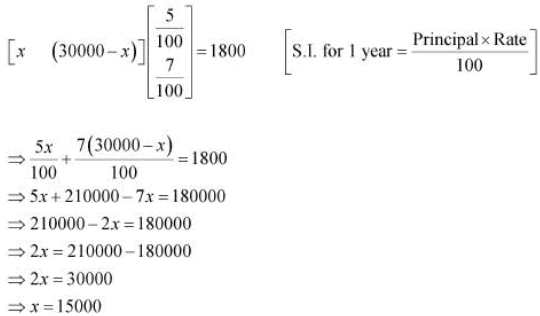Thus, in order to obtain an annual total interest of Rs 1800, the trust fund should invest
Rs 15000 in the first bond and the remaining Rs 15000 in the second bond. (b) Let Rs
x be invested in the first bond. Then, the sum of money invested in the second bond
will be Rs (30000 − x).
Therefore, in order to obtain an annual total interest of Rs 2000, we have: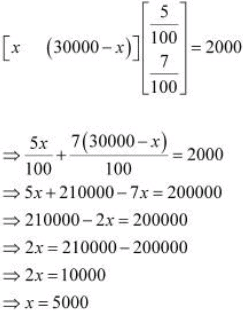Thus, in order to obtain an annual total interest of Rs 2000, the trust fund should invest
Rs 5000 in the first bond and the remaining Rs 25000 in the second bond.

Question 20:
The bookshop of a particular school has 10 dozen chemistry books, 8 dozen physics books,
10 dozen economics books. Their selling prices are Rs 80, Rs 60 and Rs 40 each
respectively. Find the total amount the bookshop will receive from selling all the books
using matrix algebra.

The bookshop has 10 dozen chemistry books, 8 dozen physics books, and 10 dozen
economics books.
The selling prices of a chemistry book, a physics book, and an economics book are
respectively given as Rs 80, Rs 60, and Rs 40.
The total amount of money that will be received from the sale of all these books can be
represented in the form of a matrix as: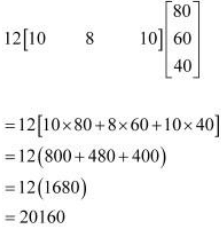Thus, the bookshop will receive Rs 20160 from the sale of all these books.

Question 21: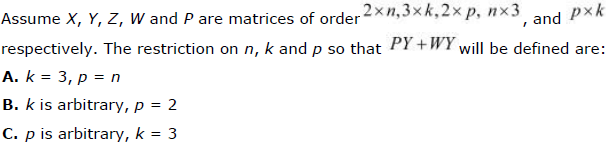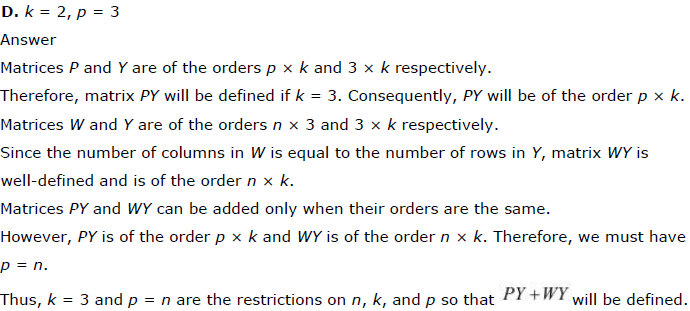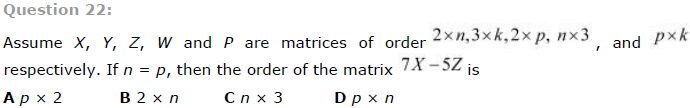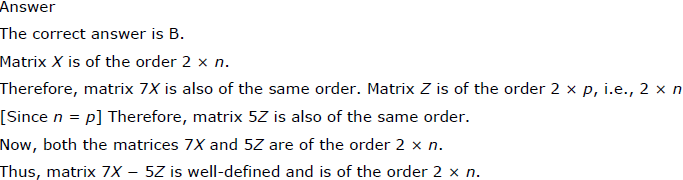The document NCERT Solutions, Matrices, Part - 2, Class 12, Maths Notes - Class 12 is a part of Class 12 category.
All you need of Class 12 at this link: Class 12Use Code STAYHOME200 and get INR 200 additional OFF

Track your progress, build streaks, highlight & save important lessons and more!

,

,

,

,

,

,

,

,

,

,

,

,

,

,

,

,

,

,

,

,

,

,

,

,

,

,

,

,

,

,

,

,

,

;